# Stripmap Synthetic Aperture Radar (SAR) Image Formation

This example shows how to model a stripmap-based Synthetic Aperture Radar (SAR) system using a Linear FM (LFM) Waveform. SAR is a type of side-looking airborne radar where the achievable cross-range resolution is much higher as compared to a real aperture radar. The image generated using SAR has its own advantages primarily pertaining to the use of an active sensor (radar) as opposed to conventional imaging systems employing a passive sensor (camera) that rely on the ambient lighting to obtain the image. Since an active sensor is used, the system provides all-weather performance irrespective of snow, fog, or rain, for example. Also, configuring the system to work at different frequencies such as L-, S-, or C-band can help analyze different layers on the ground based on varying depth of penetrations. Because the resolution of SAR depends upon the signal and antenna configuration, resolution can be much higher than for vision-based imaging systems. Using stripmap mode, this example performs both a range migration algorithm  and an approximate form of a back-projection algorithm  to image stationary targets. The approximate form of the back-projection algorithm has been chosen for the reduced computational complexity as indicated in . A Linear FM waveform offers the advantage of large time-bandwidth product at a considerably lower transmit power making it suitable for use in airborne systems.

SAR generates a two-dimensional (2-D) image. The direction of flight is referred to as the cross-range or azimuth direction. The direction of the antenna boresight (broadside) is orthogonal to the flight path and is referred to as the cross-track or range direction. These two directions provide the basis for the dimensions required to generate an image obtained from the area within the antenna beam-width through the duration of the data collection window. The cross-track direction is the direction in which pulses are transmitted. This direction provides the slant range to the targets along the flight path. The energy received after reflection off the targets for each pulse must then be processed (for range measurement and resolution). The cross-range or azimuth direction is the direction of the flight path and it is meaningful to process the ensemble of the pulses received over the entire flight path in this direction to achieve the required measurement and resolution. Correct focusing in both the directions implies a successful generation of image in the range and cross-range directions. It is a requirement for the antenna beam-width to be wide enough so that the target is illuminated for a long duration by the beam as the platform moves along its trajectory. This will help provide more phase information. The key terms frequently encountered when working with SAR are:

1. Cross-range (azimuth): this parameter defines the range along the flight path of the radar platform.

2. Range: this parameter defines the range orthogonal to the flight path of the radar platform.

3. Fast-time: this parameter defines the time duration for operation of each pulse.

4. Slow-time: this parameter defines the cross-range time information. The slow time typically defines the time instances at which the pulses are transmitted along the flight path.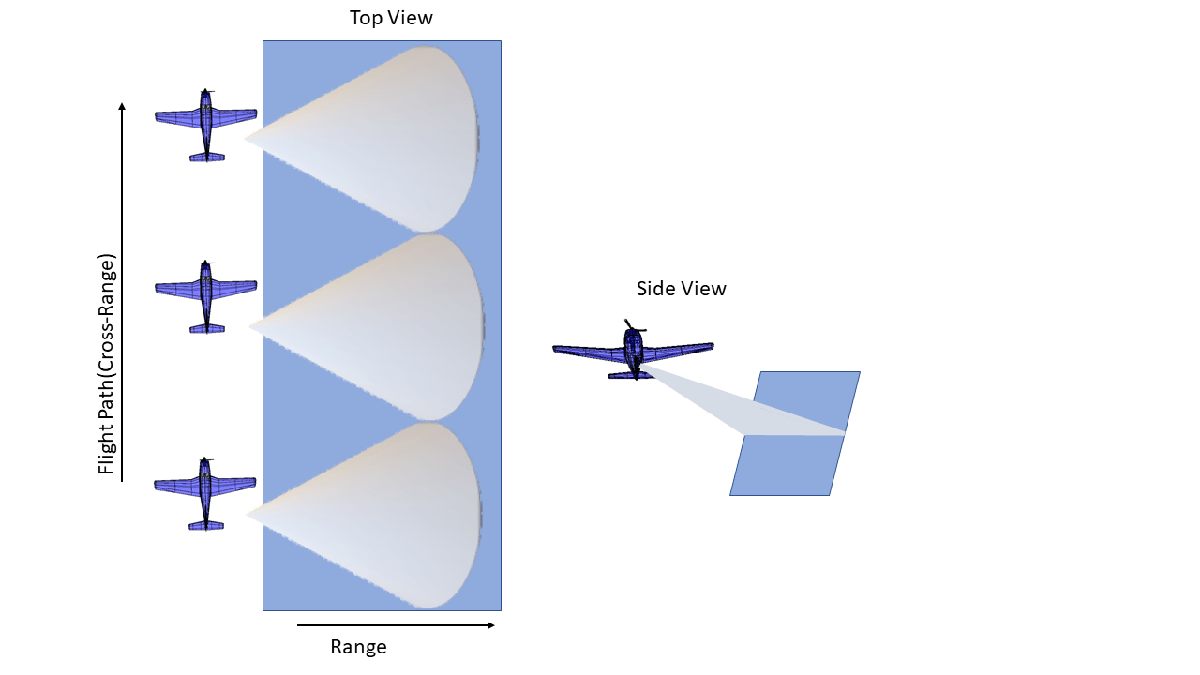Consider a SAR radar operating in C-band with a 4 GHz carrier frequency and a signal bandwidth of 50 MHz. This bandwidth yields a range resolution of 3 meters. The radar system collects data orthogonal to the direction of motion of the platform as shown in the figure above. The received signal is a delayed replica of the transmitted signal. The delay corresponds in general to the slant range between the target and the platform. For a SAR system, the slant range will vary over time as the platform traverses a path orthogonal to the direction of antenna beam. This section below focuses on defining the parameters for the transmission waveform. The LFM sweep bandwidth can be decided based on the desired range resolution.

Set the physical constant for speed of light.

`c = physconst('LightSpeed');`

Set the SAR center frequency.

`fc = 4e9;`

Set the desired range and cross-range resolution to 3 meters.

```rangeResolution = 3; crossRangeResolution = 3;```

The signal bandwidth is a parameter derived from the desired range resolution.

`bw = c/(2*rangeResolution);`

In a SAR system the PRF has dual implications. The PRF not only determines the maximum unambiguous range but also serves as the sampling frequency in the cross-range direction. If the PRF is too low to achieve a higher unambiguous range, there is a longer duration pulses resulting in fewer pulses in a particular region. At the same time, if the PRF is too high, the cross-range sampling is achieved but at the cost of reduced range. Therefore, the PRF should be less than twice the Doppler frequency and should also satisfy the criteria for maximum unambiguous range

```prf = 1000; aperture = 4; tpd = 3*10^-6; fs = 120*10^6;```

Configure the LFM signal of the radar.

```waveform = phased.LinearFMWaveform('SampleRate',fs, 'PulseWidth', tpd, 'PRF', prf,... 'SweepBandwidth', bw);```

Assume the speed of aircraft is 100 m/s with a flight duration of 4 seconds.

```speed = 100; flightDuration = 4; radarPlatform = phased.Platform('InitialPosition', [0;-200;500], 'Velocity', [0; speed; 0]); slowTime = 1/prf; numpulses = flightDuration/slowTime +1; maxRange = 2500; truncrangesamples = ceil((2*maxRange/c)*fs); fastTime = (0:1/fs:(truncrangesamples-1)/fs); % Set the reference range for the cross-range processing. Rc = 1000;```

Configure the SAR transmitter and receiver. The antenna looks in the broadside direction orthogonal to the flight direction.

```antenna = phased.CosineAntennaElement('FrequencyRange', [1e9 6e9]); antennaGain = aperture2gain(aperture,c/fc); transmitter = phased.Transmitter('PeakPower', 50e3, 'Gain', antennaGain); radiator = phased.Radiator('Sensor', antenna,'OperatingFrequency', fc, 'PropagationSpeed', c); collector = phased.Collector('Sensor', antenna, 'PropagationSpeed', c,'OperatingFrequency', fc); receiver = phased.ReceiverPreamp('SampleRate', fs, 'NoiseFigure', 30);```

Configure the propagation channel.

```channel = phased.FreeSpace('PropagationSpeed', c, 'OperatingFrequency', fc,'SampleRate', fs,... 'TwoWayPropagation', true);```

### Scene Configuration

In this example, three static point targets are configured at locations specified below. All targets have a mean RCS value of 1 meter-squared.

```targetpos= [800,0,0;1000,0,0; 1300,0,0]'; targetvel = [0,0,0;0,0,0; 0,0,0]'; target = phased.RadarTarget('OperatingFrequency', fc, 'MeanRCS', [1,1,1]); pointTargets = phased.Platform('InitialPosition', targetpos,'Velocity',targetvel); % The figure below describes the ground truth based on the target % locations. figure(1);h = axes;plot(targetpos(2,1),targetpos(1,1),'*g');hold all;plot(targetpos(2,2),targetpos(1,2),'*r');hold all;plot(targetpos(2,3),targetpos(1,3),'*b');hold off; set(h,'Ydir','reverse');xlim([-10 10]);ylim([700 1500]); title('Ground Truth');ylabel('Range');xlabel('Cross-Range');```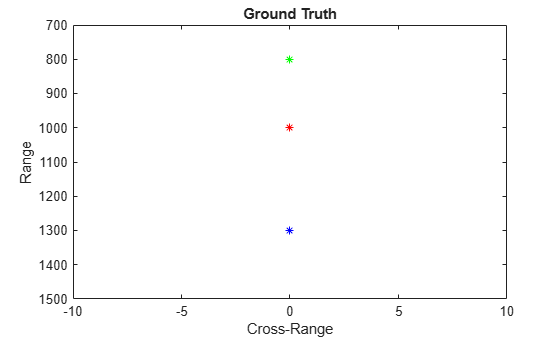### SAR Signal Simulation

The following section describes how the system operates based on the above configuration. Specifically, the section below shows how the data collection is performed for a SAR platform. As the platform moves in the cross-range direction, pulses are transmitted and received in directions orthogonal to the flight path. A collection of pulses gives the phase history of the targets lying in the illumination region as the platform moves. The longer the target lies in the illumination region, the better the cross-range resolution for the entire image because the process of range and cross-range focusing is generalized for the entire scene.

```% Define the broadside angle refangle = zeros(1,size(targetpos,2)); rxsig = zeros(truncrangesamples,numpulses); for ii = 1:numpulses % Update radar platform and target position [radarpos, radarvel] = radarPlatform(slowTime); [targetpos,targetvel] = pointTargets(slowTime); % Get the range and angle to the point targets [targetRange, targetAngle] = rangeangle(targetpos, radarpos); % Generate the LFM pulse sig = waveform(); % Use only the pulse length that will cover the targets. sig = sig(1:truncrangesamples); % Transmit the pulse sig = transmitter(sig); % Define no tilting of beam in azimuth direction targetAngle(1,:) = refangle; % Radiate the pulse towards the targets sig = radiator(sig, targetAngle); % Propagate the pulse to the point targets in free space sig = channel(sig, radarpos, targetpos, radarvel, targetvel); % Reflect the pulse off the targets sig = target(sig); % Collect the reflected pulses at the antenna sig = collector(sig, targetAngle); % Receive the signal rxsig(:,ii) = receiver(sig); end```

The received signal can now be visualized as a collection of multiple pulses transmitted in the cross-range direction. The plots show the real part of the signal for the three targets. The range and cross-range chirps can be seen clearly. The target responses can be seen as overlapping as the pulse-width is kept longer to maintain average power.

```imagesc(real(rxsig));title('SAR Raw Data') xlabel('Cross-Range Samples') ylabel('Range Samples')```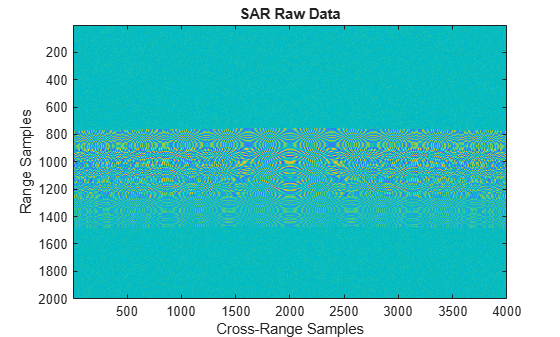Perform range compression.

Each row of the received signal, which contains all the information from each pulse, can be matched filtered to get the de-chirped/range compressed signal.

```pulseCompression = phased.RangeResponse('RangeMethod', 'Matched filter', 'PropagationSpeed', c, 'SampleRate', fs); matchingCoeff = getMatchedFilter(waveform); [cdata, rnggrid] = pulseCompression(rxsig, matchingCoeff);```

The figure below shows the response after matched filtering has been performed on the received signal. The phase histories of the three targets are clearly visible along the cross-range direction and range focusing has been achieved.

```imagesc(real(cdata));title('SAR Range Compressed Data') xlabel('Cross-Range Samples') ylabel('Range Samples')```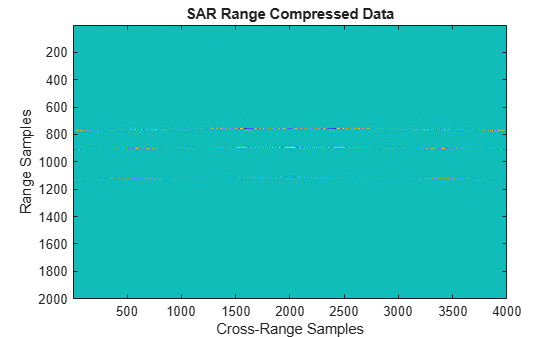Azimuth Compression

There are multiple techniques to process the cross-range data and get the final image from the SAR raw data once range compression has been achieved. In essence, the range compression helps achieve resolution in the fast-time or range direction and the resolution in the cross-range direction is achieved by azimuth or cross-range compression. Two such techniques are the range migration algorithm and the back-projection algorithm which have been demonstrated in this example.

```rma_processed = helperRangeMigration(cdata,fastTime,fc,fs,prf,speed,numpulses,c,Rc); bpa_processed = helperBackProjection(cdata,rnggrid,fastTime,fc,fs,prf,speed,crossRangeResolution,c);```

Visualize the final SAR image.

Plot the focused SAR image using the range migration algorithm and the approximate back projection algorithm. Only a section of the image formed via the range migration algorithm is shown to accurately point the location of the targets.

The range migration and accurate form of the backprojection algorithm as shown by  and  provides theoretical resolution in the cross-track as well as along-track direction. Since the back-projection used here is of the approximate form, the spread in azimuth direction is evident in case of the back-projection whereas the data processed via range migration algorithm shows that theoretical resolution is achieved.

```figure(1); imagesc((abs((rma_processed(1700:2300,600:1400).')))); title('SAR Data focused using Range Migration algorithm ') xlabel('Cross-Range Samples') ylabel('Range Samples')```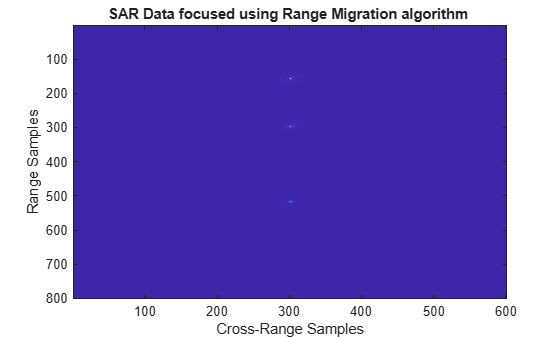```figure(2) imagesc((abs(bpa_processed(600:1400,1700:2300)))); title('SAR Data focused using Back-Projection algorithm ') xlabel('Cross-Range Samples') ylabel('Range Samples')```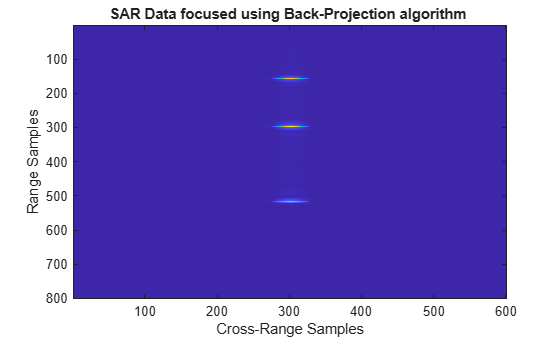### Summary

This example shows how to develop SAR processing leveraging a LFM signal in an airborne data collection scenario. The example also shows how to generate an image from the received signal via range migration and approximate form of the back-projection algorithms.

### References

1. Cafforio, C., Prati, C. and Rocca, F., 1991. SAR data focusing using seismic migration techniques. IEEE transactions on aerospace and electronic systems, 27(2), pp.194-207.

2. Cumming, I., Bennett, J., 1979. Digital Processing of Seasat SAR data. IEEE International Conference on Acoustics, Speech, and Signal Processing.

3. Na Y., Lu Y., Sun H.,A Comparison of Back-Projection and Range Migration Algorithms for Ultra-Wideband SAR Imaging, Fourth IEEE Workshop on Sensor Array and Multichannel Processing, 2006., Waltham, MA, 2006, pp. 320-324.

4. Yegulalp, A.F., 1999. Fast backprojection algorithm for synthetic aperture radar. Proceedings of the 1999 IEEE Radar Conference.

### Appendix

Range Migration Algorithm

`function azcompresseddata = helperRangeMigration(sigData,fastTime,fc,fs,prf,speed,numPulses,c,Rc)`

This function demonstrates the range migration algorithm for imaging the side looking synthetic aperture radar.The pulsed compressed synthetic aperture data is considered in this algorithm.

Set the range frequency span.

```frequencyRange = linspace(fc-fs/2,fc+fs/2,length(fastTime)); krange = 2*(2*pi*frequencyRange)/c;```

Set the cross-range wavenumber.

`kaz = 2*pi*linspace(-prf/2,prf/2,numPulses)./speed;`

Generate a matrix of the cross-range wavenumbers to match the size of the received two-dimensional SAR signal

```kazimuth = kaz.'; kx = krange.^2-kazimuth.^2;```

Set the final wavenumber to achieve azimuth focusing.

```kx = sqrt(kx.*(kx > 0)); kFinal = exp(1i*kx.*Rc);```

Perform a two-dimensional FFT on the range compressed signal.

`sdata =fftshift(fft(fftshift(fft(sigData,[],1),1),[],2),2);`

Perform bulk compression to get the azimuth compression at the reference range. Perform filtering of the 2-D FFT signal with the new cross-range wavenumber to achieve complete focusing at the reference range and as a by-product, partial focusing of targets not lying at the reference range.

`fsmPol = (sdata.').*kFinal;`

Perform Stolt Interpolation to achieve focusing for targets that are not lying at the reference range

```stoltPol = fsmPol; for i = 1:size((fsmPol),1) stoltPol(i,:) = interp1(kx(i,:),fsmPol(i,:),krange(1,:)); end stoltPol(isnan(stoltPol)) = 1e-30; stoltPol = stoltPol.*exp(-1i*krange.*Rc); azcompresseddata = ifft2(stoltPol); end```

Back-Projection Algorithm

`function data = helperBackProjection(sigdata,rnggrid,fastTime,fc,fs,prf,speed,crossRangeResolution,c)`

This function demonstrates the time-domain back projection algorithm for imaging the side-looking synthetic aperture radar. The pulsed compressed synthetic aperture data is taken as input in this algorithm. Initialize the output matrix.

```data = zeros(size(sigdata)); azimuthDist = -200:speed/prf:200;%azimuth distance```

Limit the range and cross-range pixels being processed to reduce processing time.

```rangelims = [700 1400]; crossrangelims = [-10 10];```

Index the range grid in accordance with the range limits.

`rangeIdx = [find(rnggrid>rangelims(1), 1) find(rnggrid<rangelims(2),1,'last')];`

Index the azimuth distance in accordance with the cross-range limits.

```crossrangeIdxStart = find(azimuthDist>crossrangelims(1),1); crossrangeIdxStop = find(azimuthDist<crossrangelims(2),1,'last'); for i= rangeIdx(1):rangeIdx(2) % Iterate over the range indices % Using desired cross-range resolution, compute the synthetic aperture % length lsynth= (c/fc)* (c*fastTime(i)/2)/(2*crossRangeResolution); lsar = round(lsynth*length(azimuthDist)/azimuthDist(end)) ; % Ensure lsar is an odd number lsar = lsar + mod(lsar,2); % Construct hanning window for cross-range processing, to suppress the % azimuthal side lobes hn= hanning(lsar).'; % Iterate over the cross-range indices for j= crossrangeIdxStart:crossrangeIdxStop % azimuth distance in x direction over cross-range indices posx= azimuthDist(j); % range in y-direction over range indices posy= c*fastTime(i)/2; % initializing count to zero count= 0; % Iterate over the synthetic aperture for k= j-lsar/2 +1:j+ lsar/2 % Time delay for each of range and cross-range indices td= sqrt((azimuthDist(k)- posx)^2 + posy^2)*2/c; cell= round(td*fs) +1 ; signal = sigdata(cell,k); count= count + hn(k -(j-lsar/2))*signal *exp(1j*2*pi*fc*(td)); end % Processed data at each of range and cross-range indices data(i,j)= count; end end end```